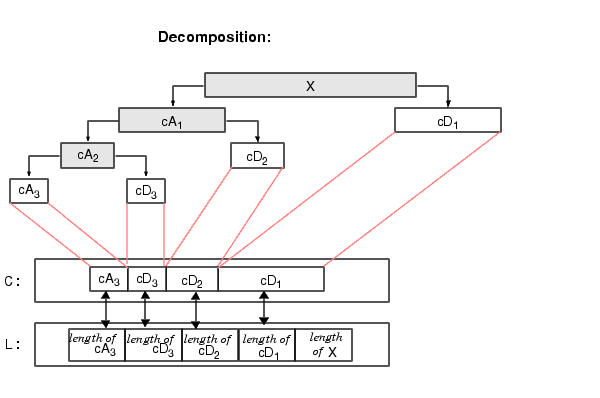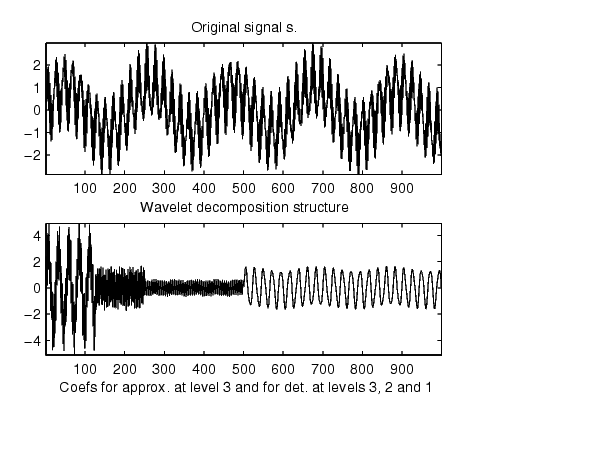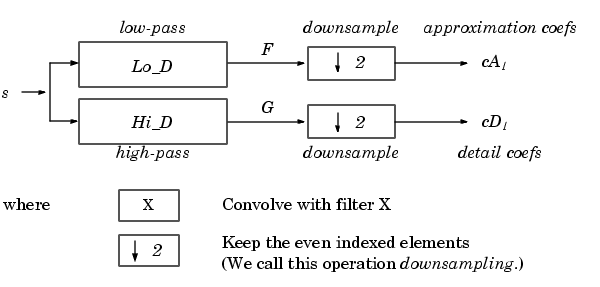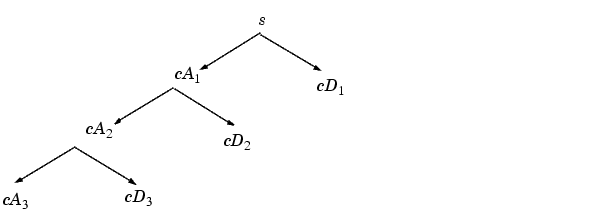Wavelet Toolboxwavedec

Multilevel 1-D wavelet decomposition

Syntax

• ```[C,L] = wavedec(X,N,'`wname`')
[C,L] = wavedec(X,N,Lo_D,Hi_D)
```

Description

`wavedec` performs a multilevel one-dimensional wavelet analysis using either a specific wavelet ('`wname`') or a specific wavelet decomposition filters (`Lo_D` and `Hi_D`, see `wfilters`).

`[C,L] = wavedec(X,N,`'`wname`'`)` returns the wavelet decomposition of the signal `X` at level `N`, using '`wname`'. `N` must be a strictly positive integer (see `wmaxlev` for more information). The output decomposition structure contains the wavelet decomposition vector `C` and the bookkeeping vector `L`. The structure is organized as in this level-3 decomposition example:`[C,L] = wavedec(X,N,Lo_D,Hi_D)` returns the decomposition structure as above, given the low- and high-pass decomposition filters you specify.

Examples

• ```% The current extension mode is zero-padding (see `dwtmode`).

% Perform decomposition at level 3 of s using db1.
[c,l] = wavedec(s,3,'db1');
% Using some plotting commands,
% the following figure is generated.```

Algorithm

Given a signal s of length N, the DWT consists of log2 N stages at most. The first step produces, starting from s, two sets of coefficients: approximation coefficients CA1, and detail coefficients CD1. These vectors are obtained by convolving s with the low-pass filter `Lo_D` for approximation, and with the high-pass filter `Hi_D` for detail, followed by dyadic decimation (downsampling).

More precisely, the first step isThe length of each filter is equal to 2N. If n = length(s), the signals F and G are of length n + 2N - 1 and the coefficients cA1 and cD1 are of length

•The next step splits the approximation coefficients cA1 in two parts using the same scheme, replacing s by cA1, and producing cA2 and cD2, and so on

.The wavelet decomposition of the signal s analyzed at level j has the following structure: [cAj, cDj, ..., cD1].

This structure contains, for J = 3, the terminal nodes of the following tree:`dwt`, `waveinfo`, `waverec`, `wfilters`, `wmaxlev`upwlev2 wavedec2